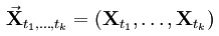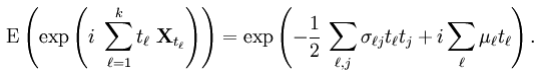Courses

# Gaussian Process Electronics and Communication Engineering (ECE) Notes | EduRev

## Communication Theory

Created by: Machine Experts

## Electronics and Communication Engineering (ECE) : Gaussian Process Electronics and Communication Engineering (ECE) Notes | EduRev

The document Gaussian Process Electronics and Communication Engineering (ECE) Notes | EduRev is a part of the Electronics and Communication Engineering (ECE) Course Communication Theory.
All you need of Electronics and Communication Engineering (ECE) at this link: Electronics and Communication Engineering (ECE)

GAUSSIAN PROCESS

In probability theory and statistics, a Gaussian process is a stochastic process whose realizations consist of random values associated with every point in a range of times (or of space) such that each such random variable has a normal distribution. Moreover, every finite collection of those random variables has a multivariate normal distribution. Gaussian processes are important in statistical modeling because of properties inherited from the normal distribution.

For example, if a random process is modeled as a Gaussian process, the distributions of various derived quantities can be obtained explicitly. Such quantities include: the average value of the process over a range of times; the error in estimating the average using sample values at a small set of times.

A process is Gaussian if and only if for every finite set of indices t1, ..., tk in the index set Tis a vector-valued Gaussian random variable. Using characteristic functions of random variables, the Gaussian property can be formulated as follows:{ Xt ; t ∈ T } is Gaussian if and only if, for every finite set of indices t1, ..., tk , there are reals σl j with σii > 0 and reals μj such thatThe numbers σlj and μj can be shown to be the covariances and means of the variables in the process.

Offer running on EduRev: Apply code STAYHOME200 to get INR 200 off on our premium plan EduRev Infinity!

,

,

,

,

,

,

,

,

,

,

,

,

,

,

,

,

,

,

,

,

,

;